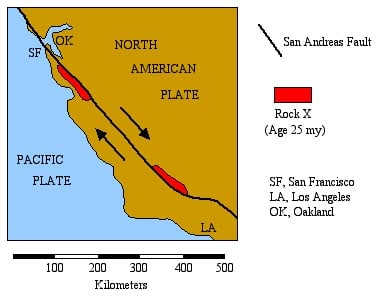SAN ANDREAS FAULT

The rate of movement along a plate boundary can be determined by measuring the displacement of rocks or other features along the boundary.  The rock marked "X" below is Miocene-Pliocene in age (~25 million years old).  The San Andreas Fault has cut through this rock unit, dividing it into two parts which are now widely separated on opposite sides of the fault.  Twenty five million years ago the two parts were formed as one body.1  What two plates are separated by the San Andreas fault?
2  Over the past 25 million years, to get to it's present position, how far has the part of rock X on the southwest side of the fault (that's the part closest to San Francisco) traveled relative to the part on the northeast side of the fault?
Movement along the San Andreas Fault has been erratic throughout it's geologic history.  Horizontal displacements of about 5 meters were recorded for the 1906 San Francisco earthquake, and such displacements are typical for major earthquakes along the fault.
3  Assuming that movements along the fault have been relatively consistent, calculate approximately how many earthquakes (at 5 meters displacement per quake) could have occurred over the past 25 million years.

Using the information given on the map, it is a simple matter to calculate the average rate of movement along the fault.  Remember, a rate of movement (velocity) can be calculated if you know the distance traveled and the time it took to make the "trip," according to the following formula:

velocity = (distance traveled) / (travel time),
or more simply, v = d / t.

4  What has been the average rate of movement along the fault over the past 25 million years, in km/million years?  in cm/year? (NOTE: 1 km = 1000 m; 1 m = 100 cm; 1 million years = 1,000,000 years.)
5  How many years will it take for Los Angeles to move up next to Oakland?